# Proofs for SMT

## Dagstuhl, October 11 2022

 Nikolaj Bjørner Microsoft Research nbjorner@microsoft.com

## Organization

• Research areas around SMT proofs

• SMT proofs in a nutshell

• A brief summary of proof efforts for SMT

• A sample of proof formats

• Proofs for EUF

• Proofs for Arithmetic

• Other proofs

• Standards?

• Directions

## Research Areas

• Connecting SMT solvers to internal/external verifiers

• Dealing with large surface areas of SMT theories

• How detailed should proofs be?

• Use proofs for profiling, learning, debugging

• Proofs for simplification vs. search

## SMT proofs in a nutshell

• Pre-processing simplification

• Proofs from CDCL(T) search

## CDCL(T) proofs

Theory solver offers two main services

## CDCL(T) proofs (II)

Both cases rely on establishing -tautologies

• tautologies are justified using theory specific rules

• tautologies can be added to propositional RUP proofs as trusted assumptions

## CDCL(T) proofs - justifying inferences

• EUF - we show how to justify EUF tautologies found by congruence closure

• Arithmetic - we illustrate extracting Farkas proofs

• Many other inferences
• Other theories - many rely on instantiating axioms.

• Trusted base (verified using ITP) using many axioms vs. trusted base with powerful checkers.

## Combining Theory Solvers

• SMT solvers combine multiple -solvers into a single solver.

• Example: EUF + Arithmetic

• For proof generation, we have a nested scenario:

• use -lemmas in CDCL solver
• combine multiple lemmas into a lemma used by the SAT solver.

## Using $T$-lemmas in CDCL

• The CDCL solver by default logs -clauses after false unit literals have been removed

• Steps required to verify -clause :

• Map to original -clause .
• Verify is a tautology
• Verify that follows from using RUP.

## Combining $T$ lemmas (I)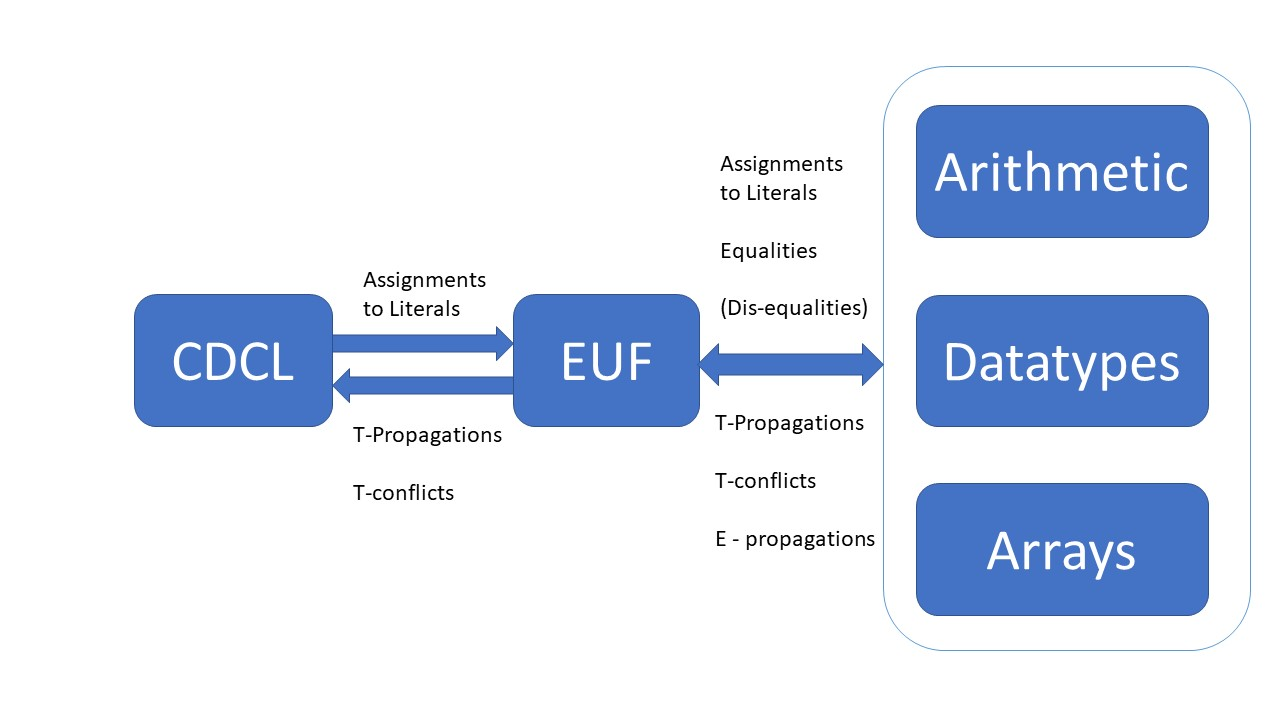T-solvers share and propagate equalities

## Combining $T$ lemmas (II)

• Each internal -propagation is logged as a lemma.

• Assumed and propagated equalities are not known by CDCL(T) solver.

• Equality reasoning leaks to -solvers

• Checking -lemmas requires equality reasoning.

## References

• Aaron Stump [1, 19, 2531]

• CVC [3, 15, 18, 23]

• SMT4J 

• SMTInterpol [16, 17]

• VeriT [2, 9]

• Z3  Axiom Profiler 

• Isabelle, Coq, LN [5, 7, 8, 11]

• Format proposals [4, 6, 24]

• SMT3 

## R - LFSC - Logical Framework with Side Conditions 

• Variant of dependent type theory with built-ins for exceptions

• Proof rules are described as dependent types
• Proof terms are type checked
• First described in 2002, then several 2008, 2013, ..

• Noteworthy:

• Integration with RUP 
• On-the-fly checking theory lemmas
• Part of CVC (Fx7, SVC) certification tool chain(s)

## R - VeriT [2, 9, 14]

• Pre-processing

• A general contextual rewrite functor

• plug in transforms for let, var, bind, app
• So far unexplored in this work:

• Global rewrites
• Fixed to post-order traversals

## R - CVC [3, 4, 15, 18, 23, 27]

• Wide set of theories

• Supported include: EUF, Arithmetic, ADT, Arrays, Strings, others
• Unsupported only: Floats, sets, sequences

• Target a variety of backends

• LFSC, LN, Coq, Isabelle
• Pre-processing proofs

## R - SMTInterpol 

• Trusted base of proof rules, see SMT Workshop 2022 paper.

• Proof rules for linear arithmetic, arrays, ADTs, EUF.

• Proof rules formulated mild extension of SMT3

• Self-contained checker

## R - Z3 - existing 

• Proof terms as expressions.
• Checks proof terms when they are created internally.
• Extract
• Isabelle tactics 
• HOL4 bit-vector proofs 
• For Z3-interpolate 
• Arithmetic proofs for SPACER

## R - Z3 - existing - limitations

• Many built-in proof term constructors. Does not parse back.
• Not easy to maintain/extend when adding new pre-processing or inference rules
• Built-in proof tooling limited to normalization for SPACER
• Many bugs related to details
• or is both a term builder and creates a clause. Sometimes a unit literal is an or.
• Proofs are optional code paths and weave many places. Forget to weave and proofs go poof.
• lack of lambda when proofs were first introduced leads to broken handling of nested quantifiers
• SPACER includes band-aid for incomplete arithmetic proof terms

## R - Z3 - RUP(T) for a new core

• Preference towards coarse-grained inferences
• Integrated self-checker
• Wishfull plans:
• trim(T)
• integrate with profiling tools (AxiomProfiler based on proof logs)
• bridges to external formats and checkers (LN?)

## F - veriT proof format 

(assume a0 (exists ((x A)) (f x)))
(anchor :step t1 :args (:= x vr))
(step t1.t1 (cl (= x vr)) :rule cong)
(step t1.t2 (cl (= (f x) (f vr))) :rule cong)
(step t1 (cl (= (exists ((x A)) (f x))
(exists ((vr A)) (f vr)))) :rule bind)
(step t2 (cl (not (= (exists ((vr A)) (f x))
(exists ((vr A)) (f vr)))) (not (exists ((vr A)) (f x)))
(exists ((vr A)) (f vr))) :rule equiv_pos1)
(step t3 (cl (exists ((vr A)) (f vr))) :premises (a0 t1 t2) :rule resolution)
(define-fun X () A (choice ((vr A)) (f vr)))
(step t4 (cl (= (exists ((vr A)) (f vr)) (f X))) :rule sko_ex)
(step t5 (cl (not (= (exists ((vr A)) (f vr)) (f X)))
(not (exists ((vr A)) (f vr))) (f X)) :rule equiv_pos1)
(step t6 (cl (f X)) :premises (t3 t4 t5) :rule resolution)

## F - CVC4 - LFSC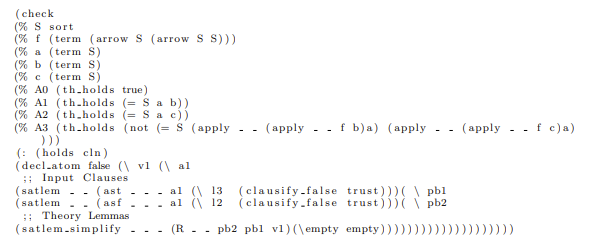## F - SMTInterpol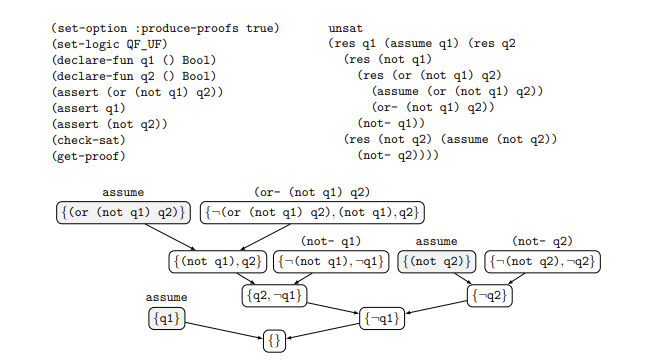## F - Z3 proof format 

(set-option :proof true)
(declare-fun f (Int) Int)
(declare-const x Int)
(assert (or (= (f (f (f x))) x) (= (f (f x)) x)))
(assert (not (= (f (f (f (f (f (f x)))))) x)))
(check-sat)
(get-proof)

## F - Z3 proof format (II)

((proof
(let ((?x25 (f x)))
(let ((?x26 (f ?x25)))
(let ((?x27 (f ?x26)))
(let ((?x32 (f ?x27)))
(let ((?x33 (f ?x32)))
(let ((?x34 (f ?x33)))
(let (($x35 (= ?x34 x))) (let (($x29 (= ?x26 x)))
(let (($x28 (= ?x27 x))) (let ((@x47 (hypothesis$x28)))
(let ((@x53 (monotonicity (monotonicity (monotonicity @x47 (= ?x32 ?x25)) (= ?x33 ?x26)) (= ?x34 ?x27))))
(let (($x36 (not$x35)))
(let ((@x37 (asserted $x36))) (let ((@x57 (lemma (unit-resolution @x37 (trans* @x53 @x47$x35) false) (not $x28)))) (let (($x30 (or $x28$x29)))
(let ((@x39 (rewrite (= $x30$x30))))
(let ((@x58 (unit-resolution (mp (mp (mp (asserted $x30) @x39$x30) @x39 $x30) @x39$x30) @x57 $x29))) (let ((@x64 (trans* (monotonicity (monotonicity @x58 (= ?x27 ?x25)) (= ?x32 ?x26)) @x58 (= ?x32 x)))) (let ((@x69 (trans* (monotonicity (monotonicity @x64 (= ?x33 ?x25)) (= ?x34 ?x26)) @x58$x35)))
(unit-resolution @x37 @x69 false))))))))))))))))))))))

## F - new Z3 proof format (II)

assume(Or(f(f(f(x))) == x, f(f(x)) == x))
assume(Not(f(f(f(f(f(f(x)))))) == x))
infer(rup, Not(f(f(f(f(f(f(x)))))) == x))
infer(euf(Not(f(f(f(f(f(f(x)))))) == x),
f(f(x)) == x,
cc(f(x) == f(f(f(x)))),
cc(f(f(f(f(x)))) == f(f(x))),
cc(f(f(f(f(f(x))))) == f(x)),
cc(f(f(f(f(f(f(x)))))) == f(f(f(f(x)))))),
Or(Not(f(f(x)) == x), f(f(f(f(f(f(x)))))) == x))
infer(rup, Not(f(f(x)) == x))
infer(rup, f(f(f(x))) == x)
infer(euf(Not(f(f(f(f(f(f(x)))))) == x),
f(f(f(x))) == x,
cc(f(x) == f(f(f(f(x))))),
cc(f(f(f(f(f(x))))) == f(f(x))),
cc(f(f(f(f(f(f(x)))))) == f(f(f(x))))),
f(f(f(f(f(f(x)))))) == x)
infer(rup, False)

## F - new Z3 proof format (III)

From SMTLIB:

(declare-fun f (Int) Int)
(define-const $24 Int (f x)) (define-const$25 Int (f $24)) (define-const$26 Int (f $25)) (define-const$27 Bool (= $26 x)) (define-const$28 Bool (= $25 x)) (assume$27 $28) (define-const$30 Int (f $26)) (define-const$31 Int (f $30)) (define-const$32 Int (f $31)) (define-const$33 Bool (= $32 x)) (assume (not$33))
(declare-fun rup () Proof)
(infer (not $33) rup) (declare-fun euf (Bool Bool Proof Proof Proof Proof) Proof) (declare-fun cc (Bool) Proof) (define-const$42 Bool (= $32$30))
(define-const $43 Proof (cc$42))
(define-const $40 Bool (=$31 $24)) (define-const$41 Proof (cc $40)) (define-const$38 Bool (= $30$25))
(define-const $39 Proof (cc$38))
(define-const $36 Bool (=$24 $26)) (define-const$37 Proof (cc $36)) (define-const$34 Bool (not $33)) (define-const$44 Proof (euf $34$28 $37$39 $41$43))
(infer (not $28)$33 $44) (infer (not$28) rup)
(infer $27 rup) (declare-fun euf (Bool Bool Proof Proof Proof) Proof) (define-const$49 Bool (= $32$26))
(define-const $50 Proof (cc$49))
(define-const $47 Bool (=$31 $25)) (define-const$48 Proof (cc $47)) (define-const$45 Bool (= $24$30))
(define-const $46 Proof (cc$45))
(define-const $51 Proof (euf$34 $27$46 $48$50))
(infer $33$51)
(infer rup)

## EUF - SMT $>$ SAT

The empty theory of first-order logic.

## EUF - Decision Procedure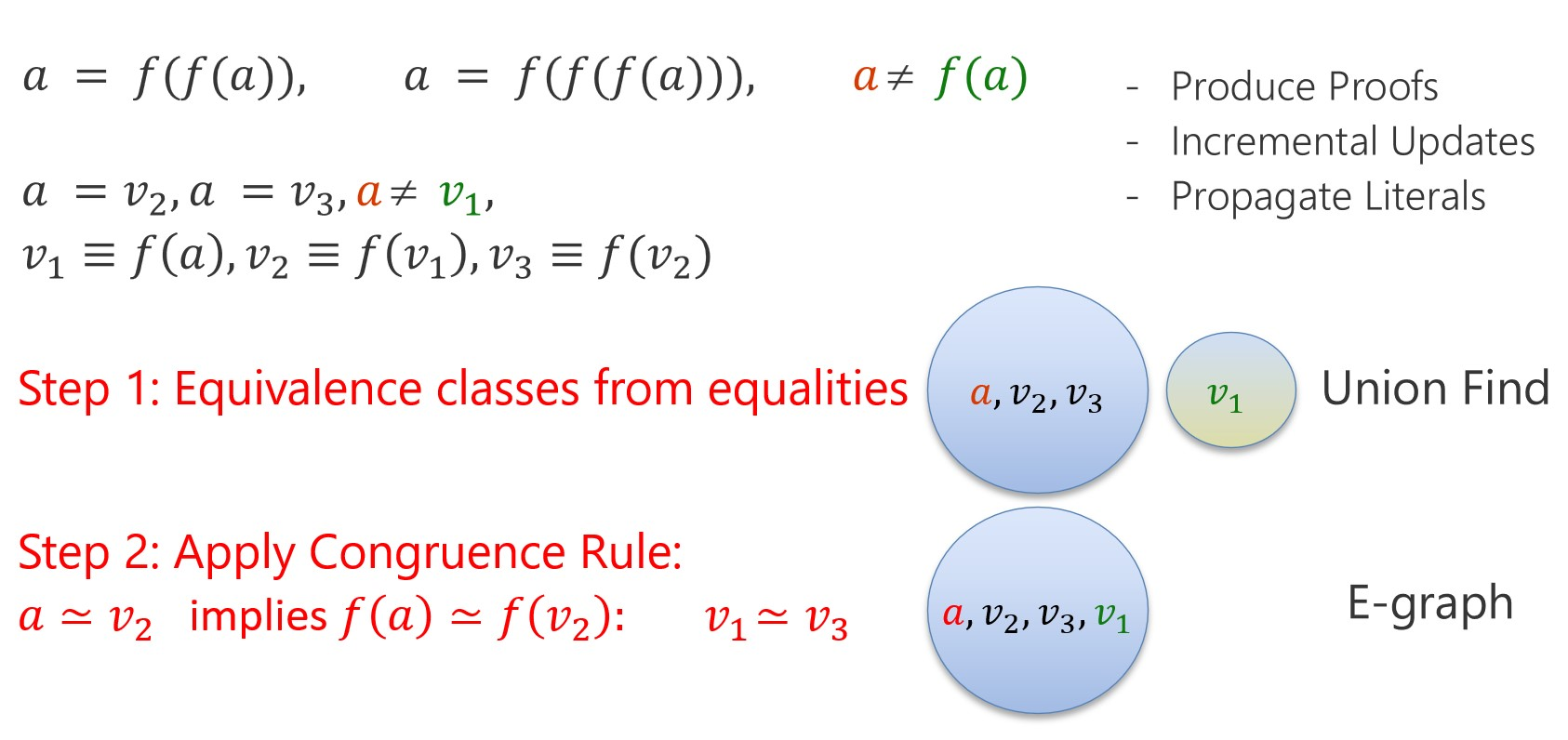## EUF - Data-structures

• E-Node:
• Union-find: - set until .
• etable:

## EUF - algorithm merge

merge()

Roots:        n1 := find(n1), r2 := find(n2)
assume (r1 != r2)
Erase:        for each p in r1.P such that p.cg == p:
erase from table
Update root:  r1.find := r2
Justify:      ....
Insert:       for each p in r1.P:
p.cg = insert p in etable
if p.cg == p:
append p to r2.P
else
add (p.cg == p) to "to_merge"


## EUF - algorithm - unmerge

unmerge():

Erase:        for each p in r2.P added from r1.P:
erase p from table
Unjustify:    ....
Revert root:  r1.find := r1
Insert:       for each p in r1.P:
insert p if n was cc root before merge

condition for being cc root before merge:
p.cg == p or !congruent(p, p.cg)

congruent(p,q) := roots of p.ts = roots of q.ts

## EUF - justifications (I)

A justification is a reason for merging two nodes. There are two possible reasons for merging nodes:

1. A literal is asserted. The justification is the literal .
2. Nodes are merged due to congruence closure.

NB: is justified recursively by justifying .

## EUF - justifications (II)

Invariant: Every non-root node points to a linked list of justifications leading to the root

## EUF - justifications (III)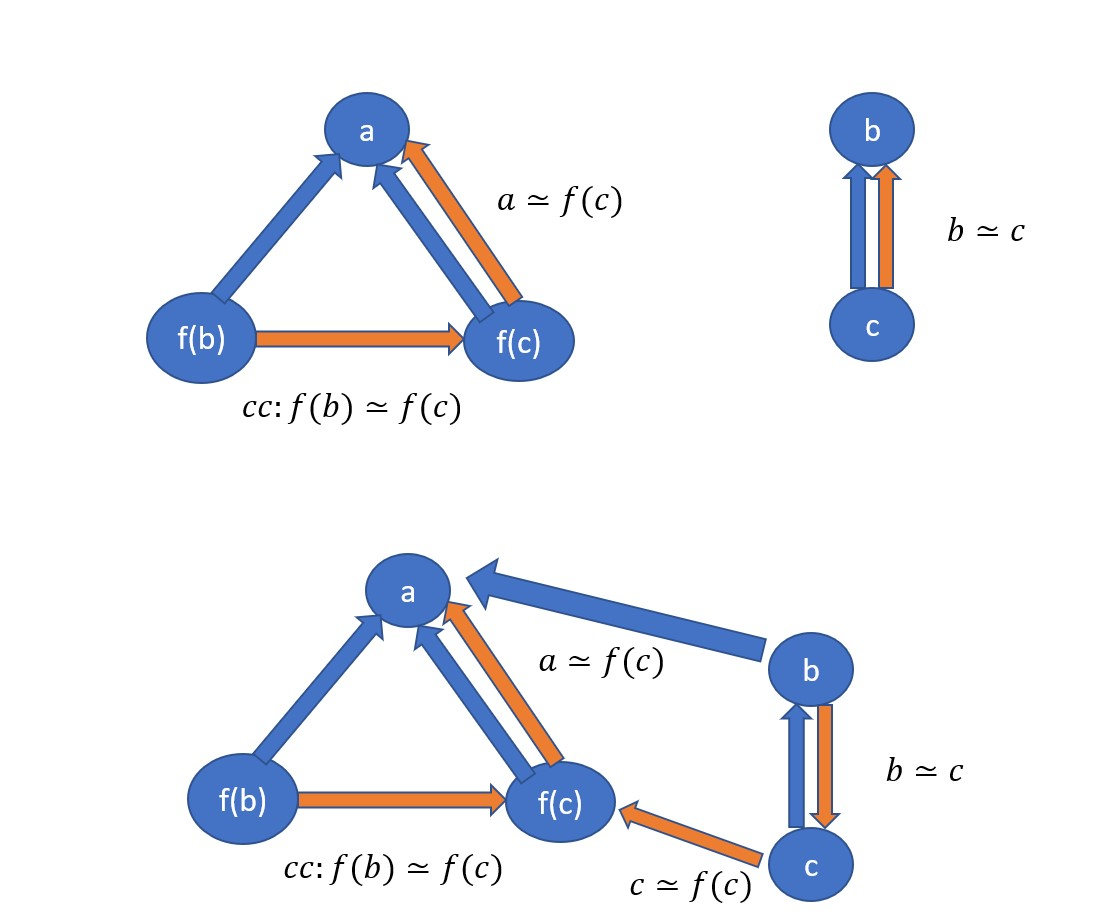## EUF - justifications are not unique

• Missed justifications:

• If then merge is a no-op.
• egg  keeps track of potential extra paths to find short proofs.

• for SMT: data-structure overhead vs. amortized effect of backtracking?

## EUF - from justifications to proofs

• Suppose follows from
• merge(), merge(),, merge().
• Proof of :

## EUF - coarse- or fine-grained proofs

• Fine-grained proofs - pro/cons:

• Proof checker is simple. Just needs to check proof rules.
• Requires building proof terms.
• produces proofs of size . Can be tuned to .
• A coarse-grained proof object (N. Shankar chat @ FLoC):

• Just list set of input literals and congruences used.
• Sort starting from leaves in (z3 sorts on a global timestamp).
• Proof checker: union-find + processing into union-find.

## EUF - Example

(set-option :sat.euf true)
(set-option :sat.smt.proof eufproof.smt2)
(declare-fun f (Int) Int)
(declare-const x Int)
(assert (or (= (f (f (f x))) x) (= (f (f x)) x)))
(assert (not (= (f (f (f (f (f (f x)))))) x)))
(check-sat)

## EUF - Example (II)

assume(Or(f(f(f(x))) == x, f(f(x)) == x))
assume(Not(f(f(f(f(f(f(x)))))) == x))
infer(rup, Not(f(f(f(f(f(f(x)))))) == x))
infer(euf(Not(f(f(f(f(f(f(x)))))) == x),
f(f(x)) == x,
cc(f(x) == f(f(f(x)))),
cc(f(f(f(f(x)))) == f(f(x))),
cc(f(f(f(f(f(x))))) == f(x)),
cc(f(f(f(f(f(f(x)))))) == f(f(f(f(x)))))),
Or(Not(f(f(x)) == x), f(f(f(f(f(f(x)))))) == x))
infer(rup, Not(f(f(x)) == x))
infer(rup, f(f(f(x))) == x)
infer(euf(Not(f(f(f(f(f(f(x)))))) == x),
f(f(f(x))) == x,
cc(f(x) == f(f(f(f(x))))),
cc(f(f(f(f(f(x))))) == f(f(x))),
cc(f(f(f(f(f(f(x)))))) == f(f(f(x))))),
f(f(f(f(f(f(x)))))) == x)
infer(rup, False)

## A - Farkas and Dual Simplex

SMT solving based on Dual Simplex maintain a tableau of the form:

where are basic and are non-basic variables.

## A - Compiling into tableau

  x, y = Reals('x y')
solve([x >= 0, Or(x + y <= 2, x + 2*y >= 6),
Or(x + y >= 2, x + 2*y > 4)])

Introduce slacks to define terms

Formula using slacks:

Only bounds (e.g., ) are asserted during search.

## A - Infeasible Tableau

A tableau is infeasible if there is a row such that

(We omit the symmetric case for upper bounds, )

The explanation for infeasibility are the literals or , respectively or .

Slack variables are unfolded into their definitions.

The justification are the coefficients

## A - Inferences

• Dual simplex infeasibility

• Bounds propagation

• Equality propagation

• Properties of div, mod, rem, to_int

• Cuts

• GCD tests

• Non-linear arithmetic

• NLSat
• Incremental Linearization
• Gröbner basis computation
• Interval reasoning

## A - Towards Generic Inferences

   (farkas c1 (<=? g1 g1') c2 (<=? g2 g2') ... cn (<=? gn gn'))
(bound c1 (<=? g1 g1') c2 (<=? g2 g2') ... cn (<=? gn gn') (not (<= e1 e2)))
(implied-eq c0 c1 (<=? g1 g1') c2 (<=? g2 g2') ... cn (<=? gn gn') (not (= e1 e2)))

where <=? is <=, <, > >=, =, and their negations; and are integer constants.

• All inferences
• Apply Gauss-Jordan elimination to equalities
• Substitute result into remaining inequalities

## A - Towards Generic Inferences - bounds

   (bound c1 (<=? g1 g1') (<=? g2 g2') ... (<=? gn gn') (not (<= e1 e2)))
• Apply Cut to the first bounds.
• Remaining inequalities are and
• Check .

## A - Towards Generic Inferences - implied equalities

   (implied-eq c0 c1 (<=? g1 g1') c2 (<=? g2 g2') ... cn (<=? gn gn') (not (= e1 e2)))

Check:

• Linear combination of first c0 inequalities implies one side of equality.
• Linear combination of remaining inequalities implies the other side.

## T - Skolem functions

Use Skolem functions, not fresh constants , when instantiating existentials

    (=> (forall ((x I)) (= (A i) (B i))) (= A B))

is instantiated as

   A = B or select(A, diff(A,B)) != select(B, diff(A, B))

Use Skolem functions to replace extended operators

(assert (contains a b))

Is rewritten into

(assert (= a (str.++ (contains.left a b) b (contains.right a b))))

## T - Hilbert Choice

   (choose ((x T)) (p x))

Used in proof generating systems (veriT, SMTInterpol).

## T - Quantifiers

• Mainstream SMT solvers are (still) Instantiation Based

## Standardization?

• SMTLIB Google Group (Hoenicke, Reynolds, others active)

• Some diversity in formats generated by provers and not clear the format from provers should/can be aligned.

## S - Farkas with SMTInterpol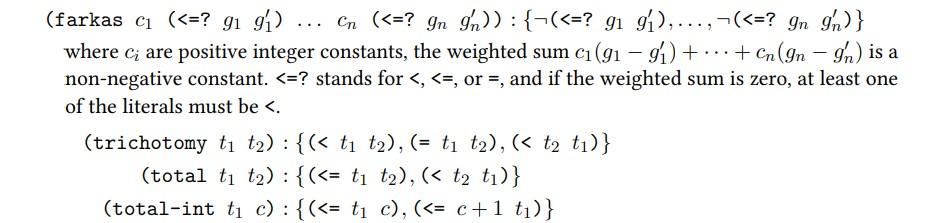## S - Farkas with Z3

   (farkas c1 (<=? g1 g1') c2 (<=? g2 g2') ... cn (<=? gn gn'))

where <=? is <=, <, > >=, =, and their negations; and are integer constants.

Define normalization function:

   ci * (>= gi gi')       ->  -ci * (<= gi gi')
ci * (< gi gi')        ->   ci * (<= (+ 1 gi) gi')        if gi is Int
ci * (> gi gi')        ->  -ci * (< gi gi')
ci * (not (<= gi gi')) ->  -ci * (< gi gi')
ci * (<= gi gi')       ->  -ci * (<= gi' gi)              if ci < 0
etc

Let be indices of inequalities, equalities, and reduce using the equalities . Then is non-negative if contains a strict inequality, or negative if it only contains weak inequalities.

## S - some impressions

• Tradeoff between generic rules and simpler rules.

• Shifts work between proof logger and proof checker
• Unclear if added complexity of checking generic rules provides return on investment.

• A proof checker could map to nucleus of SMTInterpol format before validating premises.

• Each normalization step maps to a justification

## Several Directions

• Model transformers, dual to proofs

• undo effect of model transformers for incrementality
• Pre and in-processing proofs

• proofs for global inferences
• Sustainable proofs

• proof/life balance

## Several Directions (II)

• Beyond the power of regular resolution

• for EUF
• for EPR
• Proofs for

• word-level bit-vectors
• CP domains within CDCL(T) solvers
• PB/Cardinality proofs for CDCL(T)
• Specifically extract PB justification as coarse/fine-grained proofs

## Bibliography

Andrew W. Appel, Neophytos G. Michael, Aaron Stump, and Roberto Virga. “A Trustworthy Proof Checker.” J. Autom. Reason. 31 (3-4): 231–260. 2003. doi:10.1023/B:JARS.0000021013.61329.58🔎
Haniel Barbosa, and Other. “Scalable Fine-Grained Proofs for Formula Processing.” J. Autom. Reason. 64 (3): 485–510. 2020. 🔎
Haniel Barbosa, Andrew Reynolds, Gereon Kremer, Hanna Lachnitt, Aina Niemetz, Andres Nötzli, Alex Ozdemir, et al. “Flexible Proof Production in an Industrial-Strength SMT Solver.” In Automated Reasoning - 11th International Joint Conference, IJCAR   2022, Haifa, Israel, August 8-10, 2022, Proceedings, edited by Jasmin Blanchette, Laura Kovács, and Dirk Pattinson, 13385:15–35. Lecture Notes in Computer Science. Springer. 2022. doi:10.1007/978-3-031-10769-6_3🔎
Clark W. Barrett, Leonardo Mendonça de Moura, and Pascal Fontaine. “Proofs in Satisfiability modulo Theories.” In . 2014. 🔎
Frédéric Besson, Pierre-Emmanuel Cornilleau, and David Pichardie. “Modular SMT Proofs for Fast Reflexive Checking Inside Coq.” In Certified Programs and Proofs, edited by Jean-Pierre Jouannaud and Zhong Shao, 151–166. Springer Berlin Heidelberg, Berlin, Heidelberg. 2011. 🔎
Frédéric Besson, Pascal Fontaine, and Laurent Théry. “A Flexible Proof Format for SMT: A Proposal.” In PxTP 2011: First International Workshop on Proof eXchange for Theorem   Proving, Wrocław, Poland, August 1, 2011, edited by Pascal Fontaine and Aaron Stump, 15–26. 2011. https://​pxtp2011.​loria.​fr/​PxTP2011.​pdf\#​page=​19🔎
Jasmin Christian Blanchette, Sascha Böhme, and Lawrence C. Paulson. “Extending Sledgehammer with SMT Solvers.” J. Autom. Reason. 51 (1): 109–128. 2013. doi:10.1007/s10817-013-9278-5🔎
Sascha Böhme, Anthony C. J. Fox, Thomas Sewell, and Tjark Weber. “Reconstruction of Z3’s Bit-Vector Proofs in HOL4 and Isabelle/HOL.” In Certified Programs and Proofs - First International Conference, CPP   2011, Kenting, Taiwan, December 7-9, 2011. Proceedings, edited by Jean-Pierre Jouannaud and Zhong Shao, 7086:183–198. Lecture Notes in Computer Science. Springer. 2011. doi:10.1007/978-3-642-25379-9_15🔎
Thomas Bouton, Diego Caminha Barbosa De Oliveira, David Déharbe, and Pascal Fontaine. “veriT: An Open, Trustable and Efficient SMT-Solver.” In Automated Deduction - CADE-22, 22nd International Conference on Automated   Deduction, Montreal, Canada, August 2-7, 2009. Proceedings, edited by Renate A. Schmidt, 5663:151–156. Lecture Notes in Computer Science. Springer. 2009. doi:10.1007/978-3-642-02959-2_12🔎
Chad E. Brown, Mikolás Janota, and Cezary Kaliszyk. “Abstract: Challenges and Solutions for Higher-Order SMT Proofs.” In Proceedings of the 20th Internal Workshop on Satisfiability Modulo   Theories Co-Located with the 11th International Joint Conference on Automated Reasoning (IJCAR 2022) Part of the 8th Federated Logic Conference (FLoC 2022), Haifa, Israel, August 11-12, 2022, edited by David Déharbe and Antti E. J. Hyvärinen, 3185:128. CEUR Workshop Proceedings. CEUR-WS.org. 2022. http://​ceur-​ws.​org/​Vol-​3185/​abstract697.​pdf🔎
Burak Ekici, Alain Mebsout, Cesare Tinelli, Chantal Keller, Guy Katz, Andrew Reynolds, and Clark W. Barrett. “SMTCoq: A Plug-In for Integrating SMT Solvers into Coq.” In Computer Aided Verification - 29th International Conference, CAV   2017, Heidelberg, Germany, July 24-28, 2017, Proceedings, Part II, edited by Rupak Majumdar and Viktor Kuncak, 10427:126–133. Lecture Notes in Computer Science. Springer. 2017. doi:10.1007/978-3-319-63390-9_7🔎
Katalin Fazekas. “Proofs for SMT4J.” Mathesis, Linz. https://​kfazekas.​github.​io/​papers/​Fazekas-​MasterThesis-​2015.​pdf🔎
Oliver Flatt, Samuel Coward, Max Willsey, Zachary Tatlock, and Pavel Panchekha. “Small Proofs from Congruence Closure.” In FMCAD 2022. 2022. 🔎
Pascal Fontaine, Jean-Yves Marion, Stephan Merz, Leonor Prensa Nieto, and Alwen Fernanto Tiu. “Expressiveness + Automation + Soundness: Towards Combining SMT Solvers   and Interactive Proof Assistants.” In Tools and Algorithms for the Construction and Analysis of Systems,   12th International Conference, TACAS 2006 Held as Part of the Joint European Conferences on Theory and Practice of Software, ETAPS 2006, Vienna, Austria, March 25 - April 2, 2006, Proceedings, edited by Holger Hermanns and Jens Palsberg, 3920:167–181. Lecture Notes in Computer Science. Springer. 2006. doi:10.1007/11691372_11🔎
Liana Hadarean, Clark W. Barrett, Andrew Reynolds, Cesare Tinelli, and Morgan Deters. “Fine Grained SMT Proofs for the Theory of Fixed-Width Bit-Vectors.” In Logic for Programming, Artificial Intelligence, and Reasoning - 20th   International Conference, LPAR-20 2015, Suva, Fiji, November 24-28, 2015, Proceedings, edited by Martin Davis, Ansgar Fehnker, Annabelle McIver, and Andrei Voronkov, 9450:340–355. Lecture Notes in Computer Science. Springer. 2015. doi:10.1007/978-3-662-48899-7_24🔎
Jochen Hoenicke, and Tanja Schindler. “A Simple Proof Format for SMT.” In Proceedings of the 20th Internal Workshop on Satisfiability Modulo   Theories Co-Located with the 11th International Joint Conference on Automated Reasoning (IJCAR 2022) Part of the 8th Federated Logic Conference (FLoC 2022), Haifa, Israel, August 11-12, 2022, edited by David Déharbe and Antti E. J. Hyvärinen, 3185:54–70. CEUR Workshop Proceedings. CEUR-WS.org. 2022. http://​ceur-​ws.​org/​Vol-​3185/​paper9527.​pdf🔎
Jochen Hoenicke, and Tanja Schindler. “A Simple Proof Format for SMT.” In Proceedings of the 20th Internal Workshop on Satisfiability Modulo   Theories Co-Located with the 11th International Joint Conference on Automated Reasoning (IJCAR 2022) Part of the 8th Federated Logic Conference (FLoC 2022), Haifa, Israel, August 11-12, 2022, edited by David Déharbe and Antti E. J. Hyvärinen, 3185:54–70. CEUR Workshop Proceedings. CEUR-WS.org. 2022. http://​ceur-​ws.​org/​Vol-​3185/​paper9527.​pdf🔎
Guy Katz, Clark W. Barrett, Cesare Tinelli, Andrew Reynolds, and Liana Hadarean. “Lazy Proofs for DPLL(T)-Based SMT Solvers.” In 2016 Formal Methods in Computer-Aided Design, FMCAD 2016, Mountain   View, CA, USA, October 3-6, 2016, edited by Ruzica Piskac and Muralidhar Talupur, 93–100. IEEE. 2016. doi:10.1109/FMCAD.2016.7886666🔎
Robert Klapper, and Aaron Stump. “Validated Proof-Producing Decision Procedures.” Electron. Notes Theor. Comput. Sci. 125 (3): 53–68. 2005. doi:10.1016/j.entcs.2004.06.067🔎
Kenneth L. McMillan. “Interpolants from Z3 Proofs.” In International Conference on Formal Methods in Computer-Aided Design,   FMCAD ’11, Austin, TX, USA, October 30 - November 02, 2011, 19–27. 2011. http://​dl.​acm.​org/​citation.​cfm?​id=​2157661🔎
Leonardo Mendonça de Moura, and Nikolaj S. Bjørner. “Proofs and Refutations, and Z3.” In Proceedings of the LPAR 2008 Workshops, Knowledge Exchange: Automated   Provers and Proof Assistants, and the 7th International Workshop on the Implementation of Logics, Doha, Qatar, November 22, 2008, edited by Piotr Rudnicki, Geoff Sutcliffe, Boris Konev, Renate A. Schmidt, and Stephan Schulz, volume 418. CEUR Workshop Proceedings. CEUR-WS.org. 2008. http://​ceur-​ws.​org/​Vol-​418/​paper10.​pdf🔎
Duckki Oe. “Extended Abstract: Combining a Logical Framework with an RUP Checker for SMT Proofs.” In . 2011. 🔎
Alex Ozdemir, Aina Niemetz, Mathias Preiner, Yoni Zohar, and Clark W. Barrett. “DRAT-Based Bit-Vector Proofs in CVC4.” In Theory and Applications of Satisfiability Testing - SAT 2019 - 22nd   International Conference, SAT 2019, Lisbon, Portugal, July 9-12, 2019, Proceedings, edited by Mikolás Janota and Inês Lynce, 11628:298–305. Lecture Notes in Computer Science. Springer. 2019. doi:10.1007/978-3-030-24258-9_21🔎
Hans-Jörg Schurr, Mathias Fleury, Haniel Barbosa, and Pascal Fontaine. “Alethe: Towards a Generic SMT Proof Format (extended Abstract).” In Proceedings Seventh Workshop on Proof eXchange for Theorem Proving,   PxTP 2021, Pittsburg, PA, USA, July 11, 2021, edited by Chantal Keller and Mathias Fleury, 336:49–54. EPTCS. 2021. doi:10.4204/EPTCS.336.6🔎
Aaron Stump. “Checking Validities and Proofs with CVC and Flea.” Phdthesis, Stanford University, USA. 2002. https://​searchworks.​stanford.​edu/​view/​5417778🔎
Aaron Stump. “Proof Checking Technology for Satisfiability Modulo Theories.” Electron. Notes Theor. Comput. Sci. 228: 121–133. 2009. doi:10.1016/j.entcs.2008.12.121🔎
Aaron Stump, Clark W. Barrett, and David L. Dill. “Producing Proofs from an Arithmetic Decision Procedure in Elliptical   LF.” Electron. Notes Theor. Comput. Sci. 70 (2): 29–41. 2002. doi:10.1016/S1571-0661(04)80504-8🔎
Aaron Stump, and David L. Dill. “Faster Proof Checking in the Edinburgh Logical Framework.” In Automated Deduction - CADE-18, 18th International Conference on Automated   Deduction, Copenhagen, Denmark, July 27-30, 2002, Proceedings, edited by Andrei Voronkov, 2392:392–407. Lecture Notes in Computer Science. Springer. 2002. doi:10.1007/3-540-45620-1_32🔎
Aaron Stump, Duckki Oe, Andrew Reynolds, Liana Hadarean, and Cesare Tinelli. “SMT Proof Checking Using a Logical Framework.” Formal Methods Syst. Des. 42 (1): 91–118. 2013. doi:10.1007/s10703-012-0163-3🔎
Aaron Stump, and Li-Yang Tan. “The Algebra of Equality Proofs.” In Term Rewriting and Applications, 16th International Conference, RTA   2005, Nara, Japan, April 19-21, 2005, Proceedings, edited by Jürgen Giesl, 3467:469–483. Lecture Notes in Computer Science. Springer. 2005. doi:10.1007/978-3-540-32033-3_34🔎
Dinghao Wu, Andrew W. Appel, and Aaron Stump. “Foundational Proof Checkers with Small Witnesses.” In Proceedings of the 5th International ACM SIGPLAN Conference on   Principles and Practice of Declarative Programming, 27-29 August 2003, Uppsala, Sweden, 264–274. ACM. 2003. doi:10.1145/888251.888276🔎